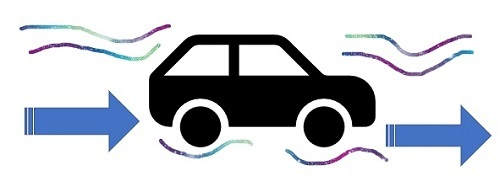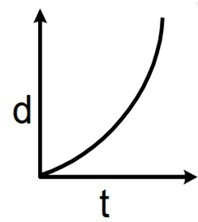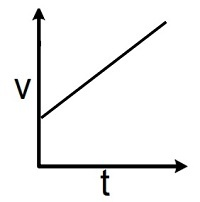# Constant Acceleration

## Introduction

Acceleration is the wrathful of all motion variables as compared to displacement and velocity. You can feel yourself accelerating in scenarios like sitting in a plane during take-off, slamming on the brakes in a car, or speeding around a corner in a go-kart.

Any procedure where the velocity varies is referred to as acceleration. There are only two ways to accelerate: changing your speed or changing your direction or changing both. This is because velocity has both a speed and a direction.## What is Constant Acceleration?

The change in velocity over time is referred to as acceleration. Constant acceleration is the state in which a body's rate of change of velocity is constant across time

Even though acceleration can be zero, positive, or negative, it is frequently used interchangeably with speeding up. When acceleration for a straight-line motion is positive, this is true. A negative acceleration in this kind of motion indicates that the body is slowing down, whereas a zero acceleration indicates that the speed remains constant.

However, acknowledging a perfect constant acceleration is exceedingly challenging. This happens because an object will always be under the impact of many forces.

For instance, if we drop a ball from the roof of a room and fall under the influence of gravity without any other force, the ball will also be subject to other atmospheric factors like air resistance. The differences in the resulting acceleration, though, might be negligible enough so that we can continue to use the idea of constant acceleration to explain its motion.

## Effect of Gravity on Constant Acceleration

All objects accelerate toward the centre of the earth due to the Earth's force of gravity. An item falling from a height accelerates almost continuously, as we've already stated. This would be a constant acceleration if we disregard the effects of air resistance and the essentially non-existent gravitational pull of other things. Additionally independent of the object's mass is the acceleration brought on by gravity.

A body that is descending from an altitude is accelerating at the frequency of g. It is possible to think of a body that is being tossed up as restricting at a rate of g before it approaches its maximum possible height, at which acceleration is zero. After reaching its highest point, the item will begin to descend and starts accelerating once more at a velocity of g.

The acceleration brought on by gravity is represented by the constant g. It is essentially equivalent to 9.8 ms-2. Unless you are given a more precise measurement, you should consider the value g = 9.8 ms-2 while dealing with problems that demand you to use acceleration due to gravity.

## Constant Acceleration on Graphs

The motion of an object can be graphically represented. In this section, we'll take a closer look at two popular graph types for illustrating the motion of an object travelling at a constant acceleration.

### Constant Acceleration in Displacement -Time Graph

The displacement-time graph can be used to depict an object's motion. On the Y-axis, displacement (d) is shown, and on the X-axis, time (t). This suggests that the change in the object's position is represented by the time it requires to get there.

• Given that velocity is the rate at which displacement changes, the gradient at any given position determines the instantaneous velocity there.

• The acceleration is zero and the velocity is constant if the displacement-time graph is a straight line.

• If the graph has a curve starting from the origin it represents that the object is under constant acceleration.

Here, we see the nature of the displacement-time graph if the object is at constant acceleration.### Constant Acceleration in Velocity-Time Graph

A velocity-time graph can also be used to depict an object's motion. Typically, the Y-axis is used to represent velocity (v), and the X-axis is used to represent time (t).

• As acceleration is the rate at which velocity changes, the gradient at a particular position on a velocity-time graph indicates the object's acceleration at that location.

• The acceleration is zero and velocity is constant if the velocity-time graph is linear.

• The region contained by the velocity-time graph and the time axis also depicts the displacement of the object if the motion is in a straight line with positive velocity.

• If the graph has a straight line, it depicts that the object is at constant acceleration. The slope of the line will give the magnitude of acceleration.## Representation of Constant Acceleration by Equations

There is a set of five widely used equations that can be used to compute five different variables for a body travelling in a specified direction with constant acceleration. The equations are referred to as the equations of constant acceleration.

• $\mathrm{S=ut+\frac{1}{2}at^2}$

• $\mathrm{S= u t-\frac{1}{2}at^2}$

• $\mathrm{S=\frac{1}{2}(u+ u)t}$

• $\mathrm{ u=u+at}$

• $\mathrm{ u^2=u^2+2as}$

## Conclusion

The variation in velocity over time is known as acceleration. Constant acceleration is the state in which a body's rate of velocity variation is constant across time.

An object's motion can be graphically represented. The two types of graphs most frequently used for this purpose are velocity-time graphs and displacement-time graphs which can also represent the motion of an object which is under constant acceleration. A body that is falling from a height is accelerating at a rate of g. (constant acceleration due to gravity). In a system with constant acceleration in a straight line, there are five frequently used equations of motion.

## FAQs

Q1. A racer starts from the rest and speeds up in a continuous uniform manner to 15 m/s in 3 s. What will the magnitude of Acceleration be?

Ans. We know that acceleration is the change in velocity.

Thus,

$$\mathrm{a=\frac{final\:velocity -initial\:velocity}{change\:in\:time}}$$

$$\mathrm{a=\frac{V_f-V_i}{\Delta t}}$$

Now, we are given that initially, the object is at rest

final velocity = 15 m/s

Time = 3 s

By formula

$$\mathrm{a=\frac{15\:m/s-0\: m/s}{3 s}}$$

$$\mathrm{a=5 m/s^2.}$$

Q2. A car traveling with velocity v=15 ms-1 after some time, the car stopped, and it started moving after 25 seconds, the car stop accelerating to attain the constant velocity at that time is v=65 ms-1. What is the magnitude of acceleration?

Ans. We have given,

Initial velocity is 15 ms-1

Final velocity is 65 ms-1

Time taken 25 seconds

Now, we know that acceleration is $\mathrm{\frac{v_f-v_i}{t_f-t_i}}$

Thus,

$$\mathrm{a=\frac{65 ms^{-1}-15 ms^{-1}}{25\:s}}$$

$$\mathrm{a=\frac{50 ms^{-1}}{25 s}=2ms^{-2}}$$

Q3. What is the example of constant acceleration?

Ans. In our daily life, we see lots of examples of constant acceleration but the most common one is the acceleration due to gravity, g. The value of g is 9.8 ms-2.

Q4. If we have a parabolic shape displacement-time graph, what type of acceleration is represented by this graph?

Ans. The parabolic shape of the displacement-time graph represents the motion of an object with constant acceleration.

Updated on: 26-Apr-2023

171 Views